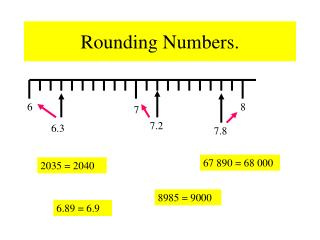DownloadDownload PresentationRounding Numbers.

# Rounding Numbers.

Télécharger la présentation## Rounding Numbers.

- - - - - - - - - - - - - - - - - - - - - - - - - - - E N D - - - - - - - - - - - - - - - - - - - - - - - - - - -
##### Presentation Transcript

1. 7.2 6.3 7.8 6 8 7 Rounding Numbers. 67 890 = 68 000 2035 = 2040 8985 = 9000 6.89 = 6.9

2. Rounded Numbers All Around You. Here are some examples of rounded numbers which could appear in newspaper headlines: 30 000 Watch Scotland Win Again. 500 000 March In War Protest. Man Leaves £2 Million To Charity. ©Microsoft Word clipart

3. 6.5 6.7 6 8 7 Rounding To The Nearest Whole Number. 6.7 Consider the number : It is our task to decide if the number is closer to : 6 7 Look at the number line below: or Mark the number 6.5 6.5 is the midpoint between 6 and 7. Mark the number 6.7 Is 6.7 closer to 6 or 7 ? Is 6.7 above or below 6.5 ? Above 6.7 = 7 (to the nearest whole number)

4. 24.3 24.5 24 26 25 Further Example. Round the following to the nearest whole number: (1) 24.3 What is the midpoint between 24 and 25 ? Is 24.3 above or below 24.5 ? Below. 24.3 = 24 (To the nearest whole number).

5. What Goes In The Box ? Round the following to the nearest whole number: NB: always round 0.5 up . Ie 12.5 = 13 (to the nearest whole number). (1) 4.8 5 (6) 287.6 288 (2) 7.4 7 (7) 9.8 10 (3) 44.6 44 (8) 49.6 50 (4) 87.5 88 (9) 99.7 100 (5) 56.3 56 (10) 399.5 400

6. 4.6 4.7 4.65 4.67 4.65 Rounding To 1 Decimal Place. Round 4.67 to one decimal place (1d.p). What number is the arrow pointing at ? Mark 4.67 on the number line . Is 4.67 closer to 4.6 or 4.7 ? 4.7 4.67 = 4.7 (to 1d.p)

7. 19.5 19.6 19.55 19.55 19.53 Round 19.53 to 1 d.p . What number is the arrow pointing at ? Mark 19.53 on the number line . Is 19.53 closer to 19.5 or 19.6 ? 19.5 19.53 = 19.5 (to 1d.p)

8. What Goes In The Box ? 2 Round the following to 1 decimal place. (1) 34.67 = 34.7 (5) 56.95 = 60 (6) 39.97 = 40 (2) 17.72 = 17.7 100 (3) 24.75 = 24.8 (7) 99.96 = (8) 999.95 = 1000 (4) 41.92 = 41.9

9. 7 Rounding To 2 Decimal Places. In order to make this a faster process we will take a different approach: Round 45.678 to 2 decimal places ( 2 d.p). What number is the second after the point ? Is the number after the seven greater or smaller than 5 ? 8 is greater than 5 (or 8 > 5) Rule If the number is greater than or equal to 5 then round up. Now round 45.678 to 2 d.p 45.678 = 45.68 (to 2 d.p)

10. 8 Round 23.482 to 2 decimal places ( 2 d.p). What number is the second after the point ? Is the number after the eight greater or smaller than 5 ? 2 is less than 5 (or 2 < 5) Rule If the number is greater than or equal to 5 then round up. Now round 23.482 to 2 d.p 23.482 = 23.48 (to 2 d.p)

11. What Goes In The Box ? 3 Round the numbers below to 2 decimal places. (1) 45.783 = 45.78 (5) 36.995 = 37 (2) 37.677 = 37.68 (6) 99.992 = 99.99 0.1 (3) 6.4589 = 6.46 (7) 0.097 = 0.43 (4) 0.4291 = (8) 999.9958 = 1000

12. 6 460 470 467 Rounding To The Nearest 10,100,1000 etc. Round 467 to the nearest ten. Which number is the tens number ? 7 > 5 Is the number after the 6 greater or smaller than 5 ? Rule If the number is greater than or equal to 5 then round up. Now round 467 to the nearest ten. 467= 470 (to the nearest ten).

13. 4 23 400 23 500 23 438 Round 23 438 to the nearest hundred. Which number is the hundreds number ? 3 < 5 Is the number after the 4 greater or smaller than 5 ? Now round 23 438 to the nearest hundred. Rule If the number is greater than or equal to 5 then round up. 23 438= 23 400 (to the nearest hundred).

14. What Goes In The Box ? 4 Round the numbers below to the required unit: (1) 34 567 to the nearest ten = 34 570 (2) 47 943 to the nearest hundred = 47 900 357 000 (3) 356 518 to the nearest thousand = (4) 356 218 to the nearest ten thousand = 360 000